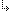- 2.2.14. -2. TMT Pascal Language Description2.2. Pascal Language Structure2.2.14. Open Arrays

## 2.2.14. Open Arrays

TMT Pascal allows one to use a multidimensional open array as parameter in procedures and functions. The open array parameter has the following format description:
`  array [dim] of type;`
where dim is a positive integer constant, defining the number of dimensions, and type is the type of the array elements. To determine the upper bounds of the array, use the High function. It returns a vector of Longints (array [0..dim-1] of Longint) containing the upper bounds. The lower bounds are always set to 0. The vector of the lower bounds can be obtained with a Low function.

Example:
```procedure print_vector (v: array (1) of Double);
var
i: integer;
begin
for i := 0 to high(v) do
Write(v[i]:10:6, ' ');
Writeln;
end;

procedure print_matrix(m: array (2) of double);
var
i: integer;
begin
for i := 0 to high(m) do
print_vector(m[i]);
Writeln;
end;

const
a: array [1..3, 1..3] of Double = ((1,0,2),(2,1,0),(1,2,1));
begin
print_matrix(a);
end.```

- 2.2.14. -